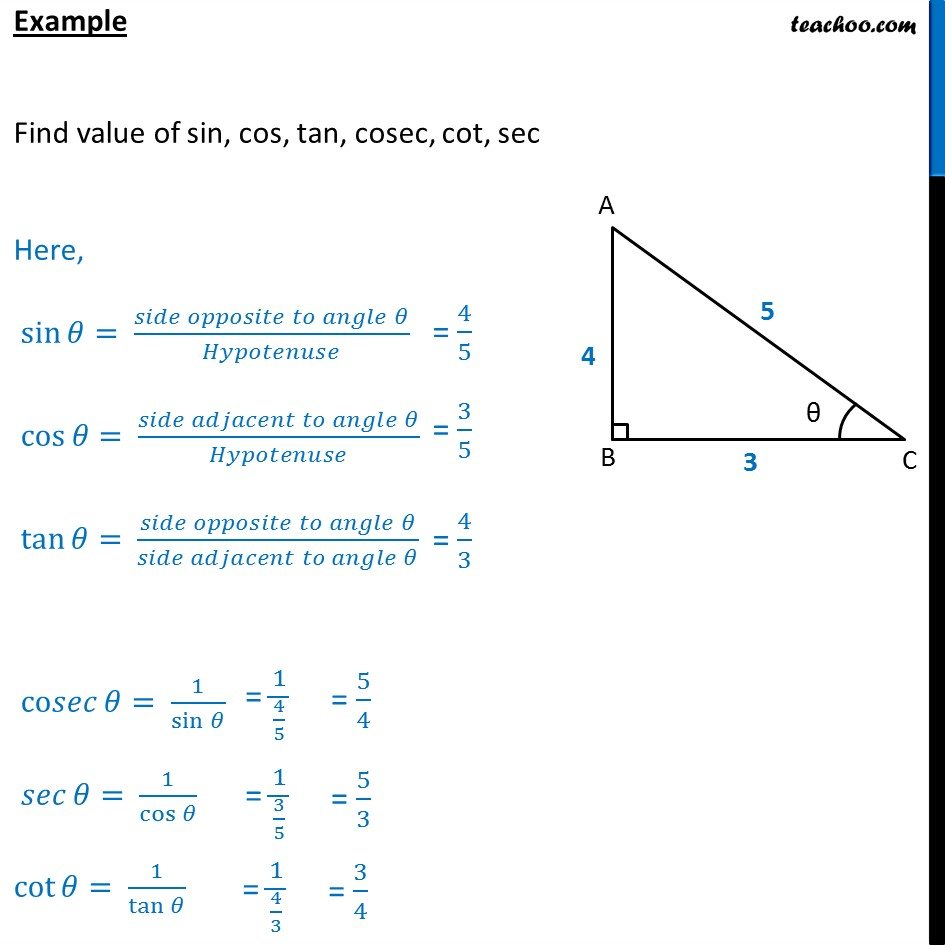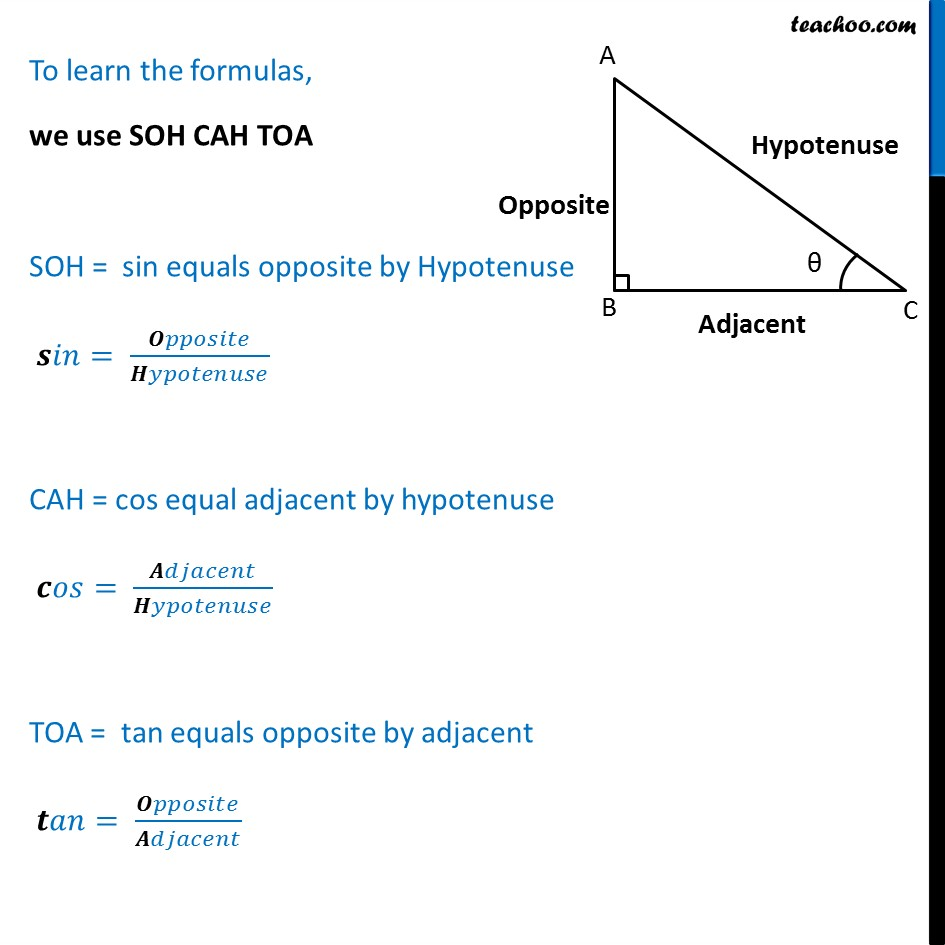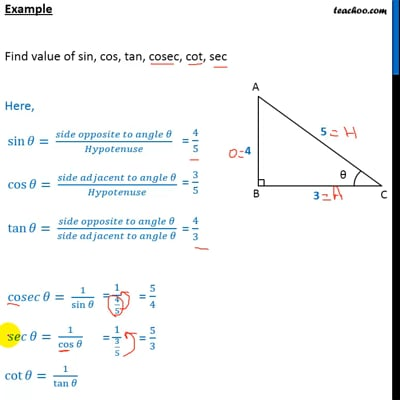Chapter 8 Class 10 Introduction to Trignometry (Term 1)
Concept wise

We will learn what sin, cos and tan are and how to find it.

## Example## SOHCAHTOAView the video in Hindi.This video is only available for Teachoo black users

### Transcript

Sin, Cos, Tan – SOH CAH TOA In right triangle ABC sin = Side opposite to angle theta / Hypotenuse cos = Side adjacent to angle theta / Hypotenuse tan = Side opposite to angle theta / Side adjacent to angle theta For cot, sec & cosec cosec = 1/ sin sec = 1/cos cot = 1/tan Example Find value of sin, cos, tan, cosec, cot, sec Given a triangle with sides 3, 4, 5 Here, Opposite side = 4 Adjacent Side = 3 Hypotenuse = 5 So, our values are sin = Side opposite to angle theta / Hypotenuse = 4/5 cos = Side adjacent to angle theta / Hypotenuse = 3/5 tan = Side opposite to angle theta / Side adjacent to angle theta = 4/3 For cot, sec & cosec cosec = 1/ sin = 5/4 sec = 1/cos = 5/3 cot = 1/tan = 3/4 To learn the formulas, we use SOH CAH TOA SOH = sin equals opposite by Hypotenuse sin = Opposite/Hypotenuse CAH = cos equal adjacent by hypotenuse cos = Adjacent/Hypotenuse TOA = tan equals opposite by adjacent tan = Opposite/Adjacent